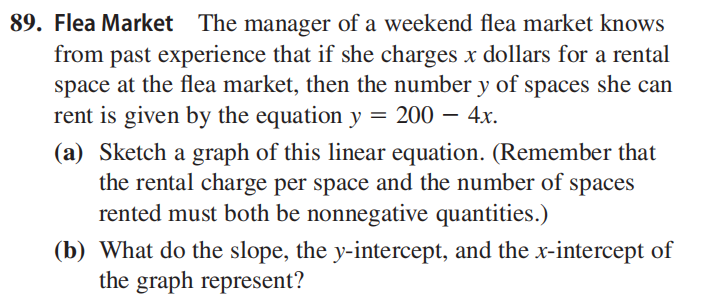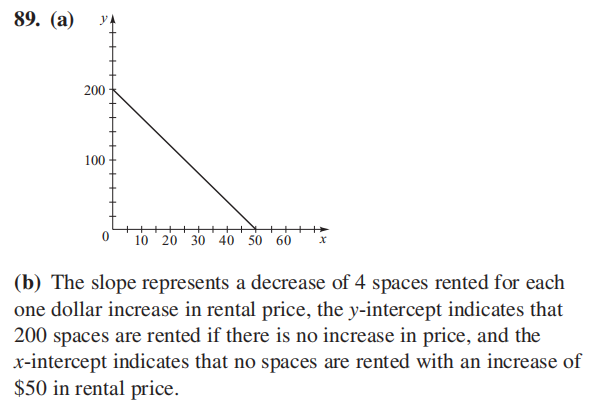### Still have math questions?

Algebra
QuestionFlea Market

The manager of a weekend flea market knows from past experience that if she charges $$x$$ dollars for a rental space at the flea market, then the number $$y$$ of spaces she can rent is given by the equation $$y = 200 - 4 x$$ .

(a) Sketch a graph of this linear equation. (Remember that the rental charge per space and the number of spaces rented must both be nonnegative quantities.)

(b) What do the slope, the $$y$$ -intercept, and the $$x$$ -intercept of the graph represent?$$\left. \begin{array} { l } { \text { 89. (a) } } \\ { \text { (b) The slope represents a decrease of } 4 \text { spaces rented for each } } \\ { \text { one dollar increase in rental price, the } y \text { - intercept indicates that } } \\ { 200 \text { spaces are rented if there is no increase in price, and the } } \\ { x \text { - intercept indicates that no spaces are rented with an increase of } } \\ { \ 50 \text { in rental price. } } \end{array} \right.$$